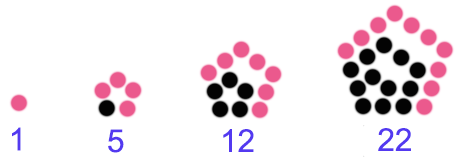# Geometric Numbers

Algebra Level 2The above shows the first 4 pentagonal numbers, namely 1, 5, 12, 22.

How many points need to be added to the $36^\text{th}$ pentagonal number to get the $37^\text{th}$ pentagonal number?

×